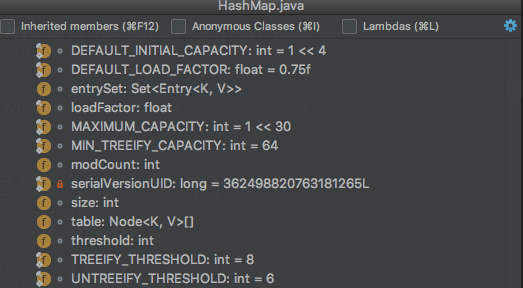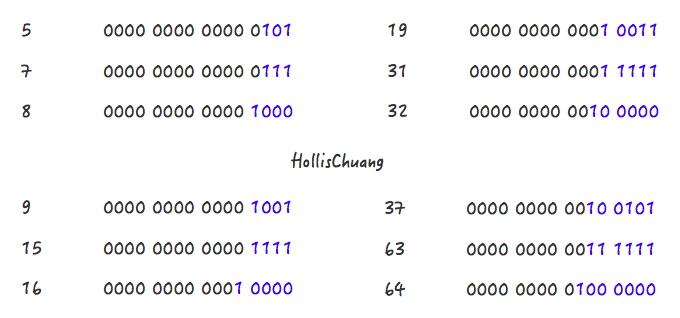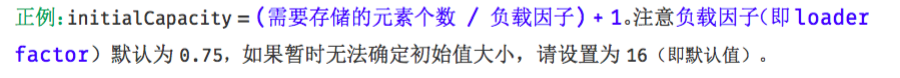HashMap中傻傻分不清楚的那些概念HashMap类中有以下主要成员变量：

• transient int size;：记录了Map中KV对的个数
• int threshold;临界值，当实际KV个数超过threshold时，HashMap会将容量扩容，threshold＝容量*加载因子
• 除了以上这些重要成员变量外，HashMap中还有一个和他们紧密相关的概念：capacity：容量，如果不指定，默认容量是16(static final int DEFAULT_INITIAL_CAPACITY = 1 << 4;)

size 和 capacity

HashMap中的size和capacity之间的区别其实解释起来也挺简单的。我们知道，HashMap就像一个“桶”，那么capacity就是这个桶“当前”最多可以装多少元素，而size表示这个桶已经装了多少元素。来看下以下代码：

Map<String, String> map = new HashMap<String, String>();
map.put("hollis", "hollischuang");

Class<?> mapType = map.getClass();
Method capacity = mapType.getDeclaredMethod("capacity");
capacity.setAccessible(true);
System.out.println("capacity : " + capacity.invoke(map));

Field size = mapType.getDeclaredField("size");
size.setAccessible(true);
System.out.println("size : " + size.get(map));

Map<String, String> map = new HashMap<String, String>(1);

Class<?> mapType = map.getClass();
Method capacity = mapType.getDeclaredMethod("capacity");
capacity.setAccessible(true);
System.out.println("capacity : " + capacity.invoke(map));

Map<String, String> map = new HashMap<String, String>(7);

Class<?> mapType = map.getClass();
Method capacity = mapType.getDeclaredMethod("capacity");
capacity.setAccessible(true);
System.out.println("capacity : " + capacity.invoke(map));

Map<String, String> map = new HashMap<String, String>(9);

Class<?> mapType = map.getClass();
Method capacity = mapType.getDeclaredMethod("capacity");
capacity.setAccessible(true);
System.out.println("capacity : " + capacity.invoke(map));

Map<String, String> map = new HashMap<>();
map.put("hollis1", "hollischuang");
map.put("hollis2", "hollischuang");
map.put("hollis3", "hollischuang");
map.put("hollis4", "hollischuang");
map.put("hollis5", "hollischuang");
map.put("hollis6", "hollischuang");
map.put("hollis7", "hollischuang");
map.put("hollis8", "hollischuang");
map.put("hollis9", "hollischuang");
map.put("hollis10", "hollischuang");
map.put("hollis11", "hollischuang");
map.put("hollis12", "hollischuang");
Class<?> mapType = map.getClass();

Method capacity = mapType.getDeclaredMethod("capacity");
capacity.setAccessible(true);
System.out.println("capacity : " + capacity.invoke(map));

Field size = mapType.getDeclaredField("size");
size.setAccessible(true);
System.out.println("size : " + size.get(map));

Field threshold = mapType.getDeclaredField("threshold");
threshold.setAccessible(true);
System.out.println("threshold : " + threshold.get(map));

map.put("hollis13", "hollischuang");
Method capacity = mapType.getDeclaredMethod("capacity");
capacity.setAccessible(true);
System.out.println("capacity : " + capacity.invoke(map));

Field size = mapType.getDeclaredField("size");
size.setAccessible(true);
System.out.println("size : " + size.get(map));

Field threshold = mapType.getDeclaredField("threshold");
threshold.setAccessible(true);
System.out.println("threshold : " + threshold.get(map));

capacity : 16
size : 12
threshold : 12

capacity : 32
size : 13
threshold : 24

总结

PS：文中分析基于JDK1.8.0_73

为什么要设置HashMap的初始化容量public static void main(String[] args) {
int aHundredMillion = 10000000;

Map<Integer, Integer> map = new HashMap<>();

long s1 = System.currentTimeMillis();
for (int i = 0; i < aHundredMillion; i++) {
map.put(i, i);
}
long s2 = System.currentTimeMillis();

System.out.println("未初始化容量，耗时 ： " + (s2 - s1));

Map<Integer, Integer> map1 = new HashMap<>(aHundredMillion / 2);

long s5 = System.currentTimeMillis();
for (int i = 0; i < aHundredMillion; i++) {
map1.put(i, i);
}
long s6 = System.currentTimeMillis();

System.out.println("初始化容量5000000，耗时 ： " + (s6 - s5));

Map<Integer, Integer> map2 = new HashMap<>(aHundredMillion);

long s3 = System.currentTimeMillis();
for (int i = 0; i < aHundredMillion; i++) {
map2.put(i, i);
}
long s4 = System.currentTimeMillis();

System.out.println("初始化容量为10000000，耗时 ： " + (s4 - s3));
}

HashMap中容量的初始化

Map<String, String> map = new HashMap<String, String>(1);
map.put("hahaha", "hollischuang");

Class<?> mapType = map.getClass();
Method capacity = mapType.getDeclaredMethod("capacity");
capacity.setAccessible(true);
System.out.println("capacity : " + capacity.invoke(map));

int n = cap - 1;
n |= n >>> 1;
n |= n >>> 2;
n |= n >>> 4;
n |= n >>> 8;
n |= n >>> 16;
return (n < 0) ? 1 : (n >= MAXIMUM_CAPACITY) ? MAXIMUM_CAPACITY : n + 1;Step 1，5->7

Step 2，7->8

Step 1，9->15

Step 2，15->16

Step 1，19->31

Step 2，31->32

n |= n >>> 1;
n |= n >>> 2;
n |= n >>> 4;
n |= n >>> 8;
n |= n >>> 16;

return (n < 0) ? 1 : (n >= MAXIMUM_CAPACITY) ? MAXIMUM_CAPACITY : n + 1;

Step 2 比较简单，就是做一下极限值的判断，然后把Step 1得到的数值+1。

Step 1 怎么理解呢？**其实是对一个二进制数依次向右移位，然后与原值取或。**其目的对于一个数字的二进制，从第一个不为0的位开始，把后面的所有位都设置成1。

1100 1100 1100 >>>1 = 0110 0110 0110
1100 1100 1100 | 0110 0110 0110 = 1110 1110 1110
1110 1110 1110 >>>2 = 0011 1011 1011
1110 1110 1110 | 0011 1011 1011 = 1111 1111 1111
1111 1111 1111 >>>4 = 1111 1111 1111
1111 1111 1111 | 1111 1111 1111 = 1111 1111 1111

Step 1:
0100 >>>1 = 0010
0100 | 0010 = 0110
0110 >>>1 = 0011
0110 | 0011 = 0111
Step 2:
0111 + 0001 = 1000

int n = cap - 1;

int n = cap - 1;
n |= n >>> 1;
n |= n >>> 2;
n |= n >>> 4;
n |= n >>> 8;
n |= n >>> 16;
return (n < 0) ? 1 : (n >= MAXIMUM_CAPACITY) ? MAXIMUM_CAPACITY : n + 1;

HashMap中初始容量的合理值public static <K, V> HashMap<K, V> newHashMapWithExpectedSize(int expectedSize) {
return new HashMap<K, V>(capacity(expectedSize));
}

/**
* Returns a capacity that is sufficient to keep the map from being resized as long as it grows no
* larger than expectedSize and the load factor is ≥ its default (0.75).
*/
static int capacity(int expectedSize) {
if (expectedSize < 3) {
checkNonnegative(expectedSize, "expectedSize");
return expectedSize + 1;
}
if (expectedSize < Ints.MAX_POWER_OF_TWO) {
// This is the calculation used in JDK8 to resize when a putAll
// happens; it seems to be the most conservative calculation we
// can make.  0.75 is the default load factor.
return (int) ((float) expectedSize / 0.75F + 1.0F);
}
return Integer.MAX_VALUE; // any large value
}

return (int) ((float) expectedSize / 0.75F + 1.0F);上面有一行注释，说明了这个公式也不是guava原创，参考的是JDK8中putAll方法中的实现的。感兴趣的读者可以去看下putAll方法的实现，也是以上的这个公式。

总结

Map<String, String> map = Maps.newHashMapWithExpectedSize(10);

• 18 min read

CONTRIBUTORS
•LiuYao

• 18 min read